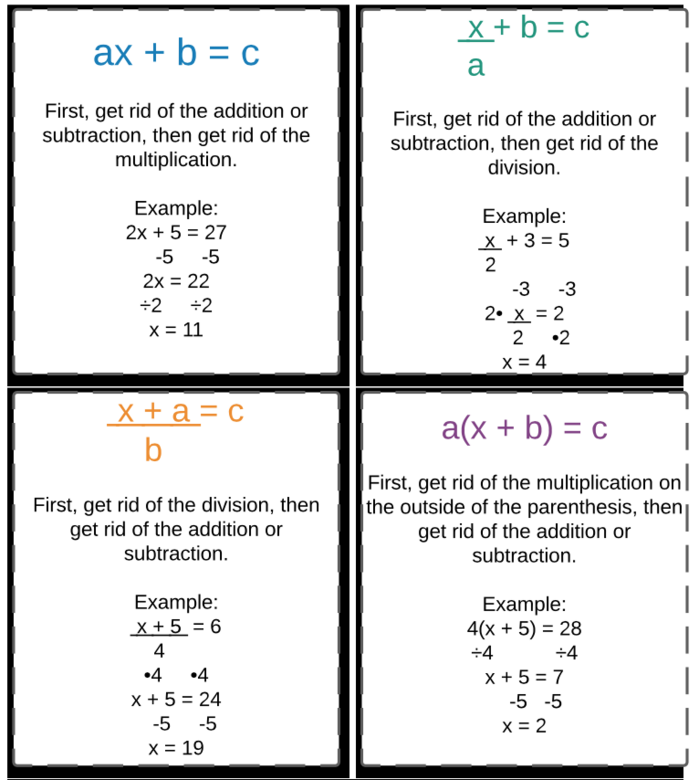Author:
Krystin
Subject:
Secondary Mathematics
Material Type:
Activity/Lab
Level:
Middle School
Tags:
Lesson Plan, Mac Apps, utah-mac-lesson
Language:
English
Media Formats:
Interactive, Text/HTML

# Solving Two-Step Equations with Integers Review## Overview

This lesson is to help students review solving two-step equations with integers. Students will create a review sheet or presentation to help them remember the different ways to solve the four types of two-step equations.

This should take about 1-2 class periods, as it is a review.

Students can complete this assignment synchronous together in a classroom, asynchronous on their own time as long as they have the Mac Applications needed.

Standard 7.EE.4: Use variables to represent quantities in a real-world or mathematical problem, and construct simple equations and inequalities to solve problems by reasoning about the quantities.

a. Solve word problems leading to equations of the form px+q=rand p(x+q)=r, where p, q, and rare specific rational numbers. Solve equations of these forms fluently. Compare an algebraic solution to an arithmetic solution, identifying the sequence of the operations used in each approach.

The only author is me, Krystin Carter-Sanchez.

# Summary

This lesson is to help students review solving two-step equations with integers. Students will create a review sheet or presentation to help them remember the different ways to solve the four types of two-step equations.

This should take about 1-2 class periods, as it is a review.

Students can complete this assignment synchronous together in a classroom, asynchronous on their own time as long as they have the Mac Applications needed.

Standard 7.EE.4: Use variables to represent quantities in a real-world or mathematical problem, and construct simple equations and inequalities to solve problems by reasoning about the quantities.

a. Solve word problems leading to equations of the form px+q=rand p(x+q)=r, where p, q, and rare specific rational numbers. Solve equations of these forms fluently. Compare an algebraic solution to an arithmetic solution, identifying the sequence of the operations used in each approach.

The only author is myself, Krystin Carter-Sanchez.

# Step 1 - Goals and Outcomes

Learning Intentions:

• Students will be able to find the solution to a two-step equation involving integers.
• Utah Math core standard 7.EE.4

Success Criteria:

• Students will accurately show and represent how to solve each of the four two-step equation models.

# Step 2 - Planning Instruction

Student Background Knowledge

Prior to this lesson, students will need to have learned the following standards:

• Solve one-step equations with integers
• Solve two-step equations with integers (all four models)

Students will do a warm up solving one of each of the two-step equations, then make a keynote presentation explaining how to solve each problem.

Strategies for Diverse Learners

Teachers can adjust this lesson to meet the needs of diverse learners by having students type their answers, having students record their answers, or having students use pages to draw their answers. Students can refer to notes taken in class as well as work with peers or the teacher to create their keynote presentation. On the assessment, students are able to type, record, or draw their answers as well.

# Step 3 - Instruction

Instructional Procedures:

To start this lesson, you will begin with a warm up of four two step equation problems:After students are finished working, go over this warm up, giving students opportunities to solve problems on their own, checking work with a neighbor as well as listening to others explain their work.

Next, students will use the Mac App Keynote to create a review of the four types of equations. They need to have the following at least:

1. An intro page with their name on it

2. An example problem of each type (they may use the warm up as example problems). For each example problem, there should be the problem, the solution, and how they found the solution. They can use pages or keynote to make graphics to show their work, they can record themselves explaining what they did, they can type their steps, or use whatever method they want to show how they found the answer.

3. They will share their keynote with the class to help review the four methods of two-step equations. There is an example attached to this section in Section Resources that shows the "perfect example" of what students should produce. Students should be creative while making this keynote presentation review.

# Step 4 - Assessments

One form of assessment in this lesson is the warm up that will happen at the beginning of class.

The other form of assessment is attached below. It is four two-step equation problems involving integers. The success criteria for this lesson is "I can solve all four types of two-step equation problems involving integers."